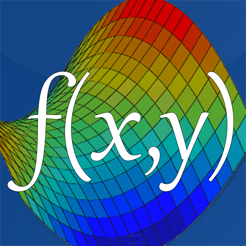## スクリーンショット

•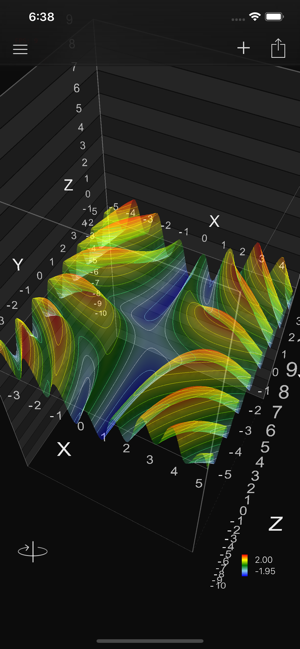•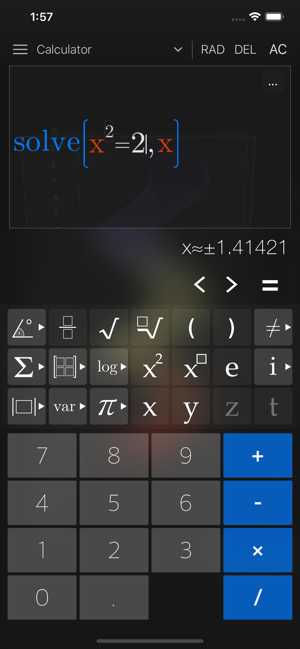•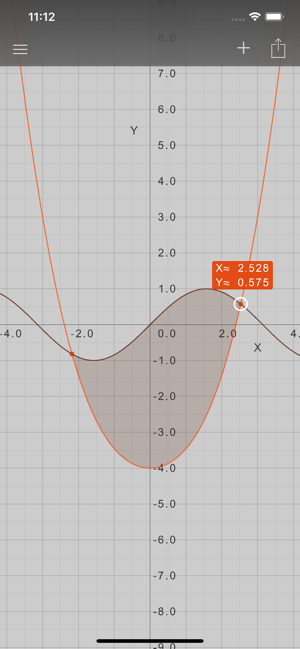•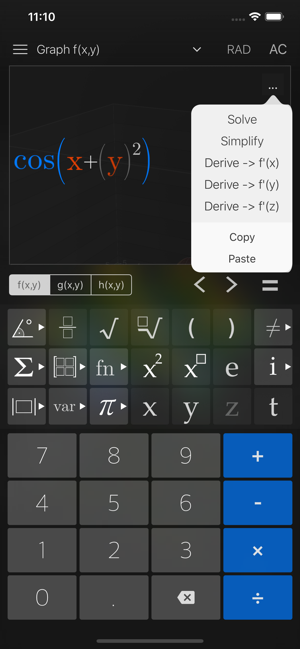•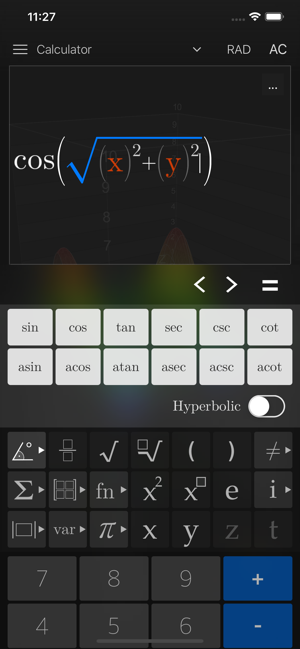•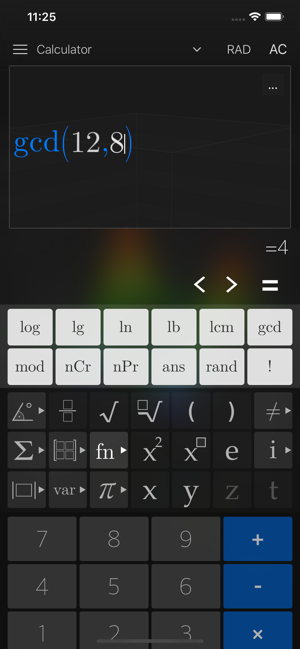•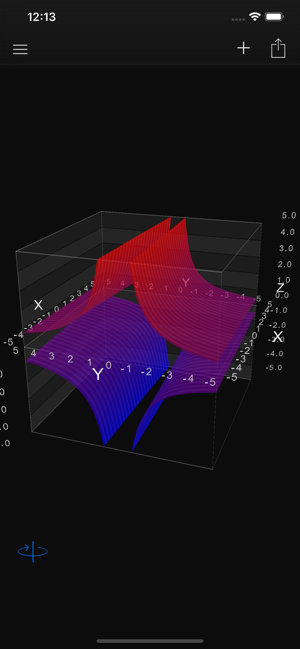•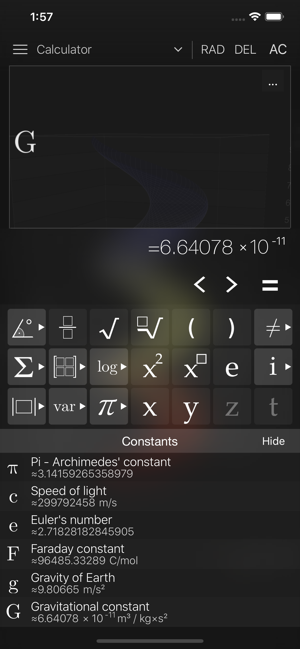•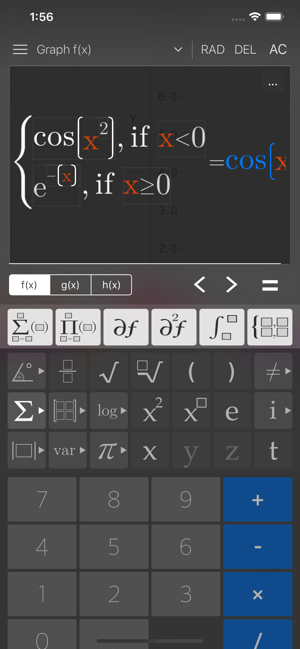•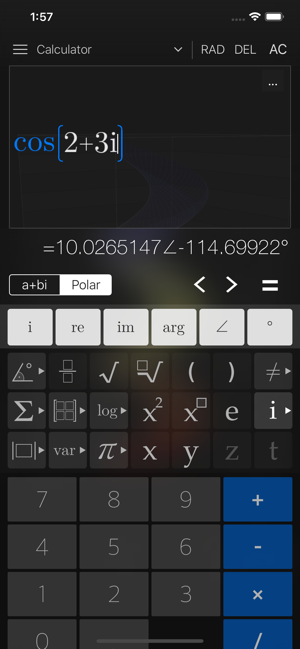## 説明

Visual Math 4D is a graphical calculator that allows you to visualize and solve your mathematical equations. It supports various types of equations, such as polar, spherical, parametric and implicit equations, which can be visualized and animated in 2D and 3D. You can also plot and animate vector fields in 2D and 3D.

Features:
- solve equations
- plot cartesian functions with intersections
- plot polar- and spherical functions
- plot parametric equations in 2D and 3D
- plot complex functions (draw real part & imaginary part)
- plot vector fields in 2D and 3D
- plot implicit equations in 2D and 3D
- plot contour maps ins 2D and 3D
- support for complex numbers
- vectors & matrices
- truth- and value tables
- trigonometrical and hyperbolic functions
- piecewise-defined functions
- logarithmic functions
- logical & binary operators
- definite integral
- n-th derivations
- statistical functions (nPr, nCr, rand..)
- physical and mathematical constants with their units
- animate variables
- share content with other apps
- no network connection required
- and a lot more

The app is easy to use and helps students and engineers to visualize and solve their mathematical equations.

## 新機能

バージョン 5.3

- draw intersection points and show their coordinates
- optimized expression rendering
- select and focus functions using gestures.
- new functions are supported, like modulo, fact, nPr, nCr, lcm, gcd, rand etc..
- support for the last result (ans)
- copy & paste your equations
- new device support

## 評価とレビュー

4.7／5
69件の評価

69件の評価

iPhoneを徹底的に活用したい!

うーん、こまった。

## 情報

Ronny Weidemann
サイズ
117.2MB
カテゴリ

4+

¥250
App内課金有り
1. Coffee ¥120
2. Cappuccino ¥250
3. Sandwich ¥610

## サポート

•